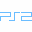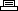Site SearchFinding NemoPrinter Friendly Version

```Level select:
Press Triangle(3), Square(2), Circle, Square, Triangle, Circle, Square,
Triangle, Square, Triangle, Square, Triangle, Circle, Triangle(2) at the title
screen, the load or start a new game. Pause game play to access the level
selection option.

Invincibility:
Press Triangle, Square(2), Circle(3), Triangle(2), Square(3), Circle(4),
Square, Triangle, Circle(3), Square,Circle, Triangle,Circle(2), Square,
Circle(2), Triangle, Circle, Square, Circle(3), Triangle at the title screen,
the load or start a new game. Note: The invincibility does not occur on all
levels. Pause game play to access the cheat option at the level selection menu.

Credits:
Press Triangle, Square, Circle, Triangle(2), Square, Circle, Triangle, Square,
Circle, Triangle, Square(2), Circle, Triangle, Square, Circle, Triangle,
Square, Circle(2), Triangle, Square, Circle at the title screen, the load or
start a new game. Pause game play to access the cheat option at the level